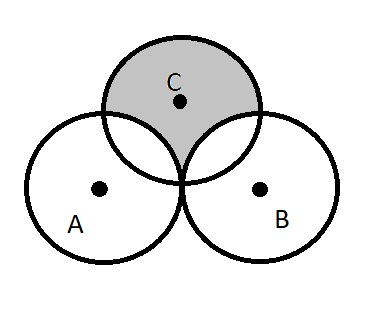# Circles A. B, and C cach have raditus 1. CirclesRupert Albert 2022-03-03 Answered
Circles A. B, and C cach have raditus 1. Circles A and B share one point of tangency. Circle C has a point of tangency with the midpoint of $\stackrel{―}{AB}$. What is the area inside circle C but outside circle A and circle B?a) $3-\frac{\pi }{2}$
b) $\frac{\pi }{2}$
c) 2
d) $\frac{3\pi }{4}$
e) $1+\frac{\pi }{2}$
You can still ask an expert for help

• Questions are typically answered in as fast as 30 minutes

Solve your problem for the price of one coffee

• Math expert for every subject
• Pay only if we can solve itPooja Copeland

Stpe 1
Given, Circles A, B, and C each have radius 1. Circles A and B share one point of tangency.
Circle C has a point of tangency with the midpoint of $\stackrel{―}{AB}$.
To find the are inside circle C but outside A and circle B, that is, the shaded region of the circle C.
Now, $\text{Area of shaded region}=\text{Area of Circle C}-\text{Area shared between Circles A, B and C}$.
Let S be the mid point of $\stackrel{―}{AB}$ and T be the point of intersection of Circles A and C.
Join the points by joining the points ST
Step 2
Now, the shared area of all three circles the region between line ST and arc ST.
The area of the Circle $A=\pi {r}^{2}=\pi$
The area of
$\mathrm{△}SAT=\frac{1}{2}bh$
$=\frac{1}{2}$ since $b=h=r=1$
So, the area between the line ST and the arc $ST=\frac{1}{4}×$ (Area of Circle A) - Area of $\mathrm{△}SAT$
$=\frac{1}{4}×\pi -\frac{1}{2}=\left(\frac{\pi -2}{4}\right)$
Step 3
Area of Shaded region in $\text{Circle C}=\text{(Area of Circle C )}-4$ (Area between the line ST and the arc ST)
$=\pi {\left(1\right)}^{2}-4\left(\frac{\pi -2}{4}\right)=\pi -\pi +2=2$
Therefore, Area of Shaded region in Circle C is 2.